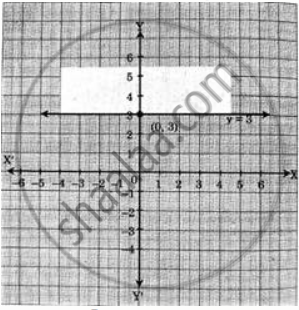# Solve the following inequation graphically in the two-dimensional plane.y ≥ 3 - Mathematics and Statistics

Graph

Solve the following inequation graphically in the two-dimensional plane.
y ≥ 3

#### Solution

Given, inequation is y ≥ 3
∴ corresponding equation is y = 3
It is a line parallel to X-axis passing through the point A(0, 3)
Origin test:
Substituting y = 0 in inequation, we get
0 ≥ 3 which is false.
∴ Points on the origin side of the line do not satisfy the inequation
∴ Points on the non-origin side of the line satisfy the inequation
∴ all the points on the line and above it satisfy the given inequation.
The shaded portion represents the solution set.Concept: Graphical Solution of Linear Inequality of Two Variable
Is there an error in this question or solution?
Chapter 8: Linear Inequations - Exercise 8.2 [Page 120]

#### APPEARS IN

Balbharati Mathematics and Statistics 2 (Commerce) 11th Standard HSC Maharashtra State Board
Chapter 8 Linear Inequations
Exercise 8.2 | Q 1. (ii) | Page 120
Share